## Egg Carton Math Games First GradeMany elementary teachers use math centers in their classrooms.  They’re fun and engaging ways to review math concepts or extend instruction.  However, it’s often time consuming to put them together, and they usually only cover one math skill.

Here’s an easy solution:  Make 22 math centers in a matter of minutes using something I’m pretty sure you have sitting in your fridge.  Let me show you!

## Egg Carton Math Games for First Grade

#### Materials:

• egg cartons
• 3 or more marbles or small round objects
• permanent marker
• small round dot labels (optional)

#### Set Up:

All of the activities require something to be written inside each egg space.  Many activities use the numbers 1-12, some use 0-9, and a few use shapes, coins, or colors.  I’ve grouped the activities below according to what numbers or symbols you use.

Use a permanent marker to write the numbers inside each egg space.  (Optional: Write the numbers on small round dot labels and place inside the egg carton.  A bit of glue or tape is needed to hold the dots in place during all the shaking!)

#### Directions:

The steps for each of the games are the same:

1. Open the egg carton.
2. Place a specified number of marbles or small round objects inside and close the lid.
3. SHAKE, SHAKE, SHAKE the egg carton all round!
4. Open the lid.  See what numbers/objects the marbles land on and perform a certain task with them.

## Egg Carton Math Games Using Numbers 1-12

###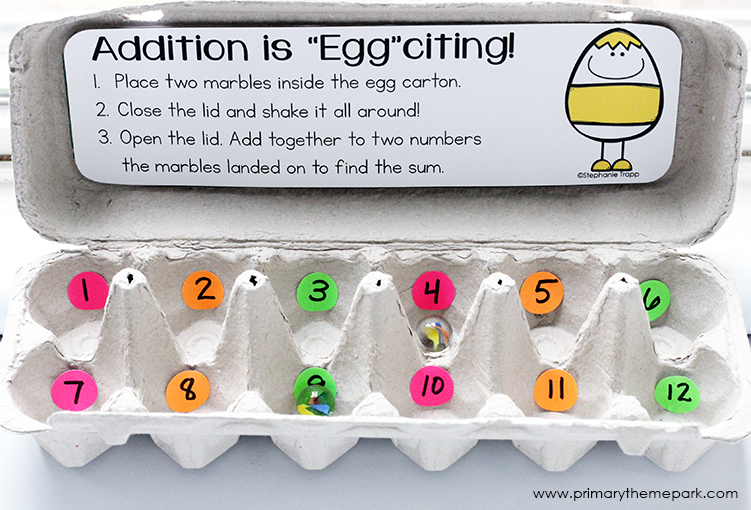Need: 2 marbles or small round objects

Add the two numbers together to find the sum. Example: 4+9=13.

### Subtraction to 20

Need: 2 marbles or small round objects

Subtract the smaller number from the larger number to find the difference.  Example: 9-4=5.

### Fact Families

Need: 2 marbles or small round objects

Write two addition and two subtraction sentences using the numbers.  Example: 9+4=13, 4+9=13, 13-9=4, 13-4=9.

### True/False Equations

Need: 2 marbles or small round objects

Write one true and one false addition or subtraction equation using the numbers the marbles land on. Example: True- 9+4=13, False- 9-4=2.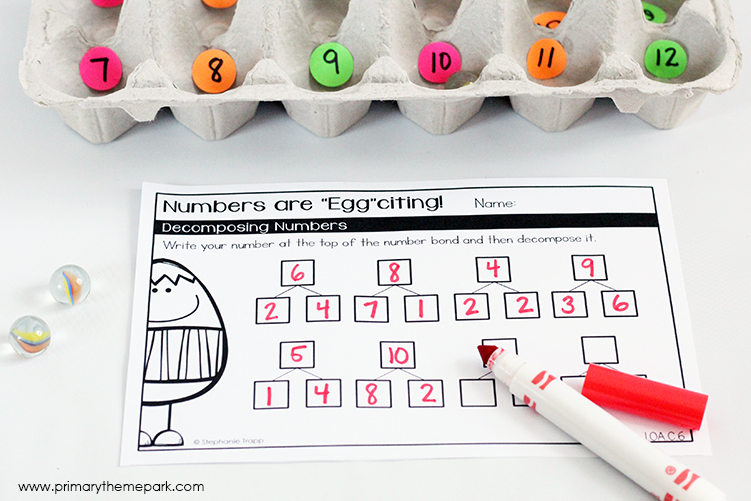### Decomposing Numbers

Need: 1 marble or small round object

Decompose the number using a number bond.

### Finding Unknown Numbers

Need: 2 marbles or small round objects

Find what number when added to the smaller number equals the larger number, or what number subtracted from the larger number equals the smaller number. Example: 4+?=9, answer is 5,  9-?=4, answer is 5.

### Doubles

Need: 1 marble or small round object

Double the number. Example: 10+10=20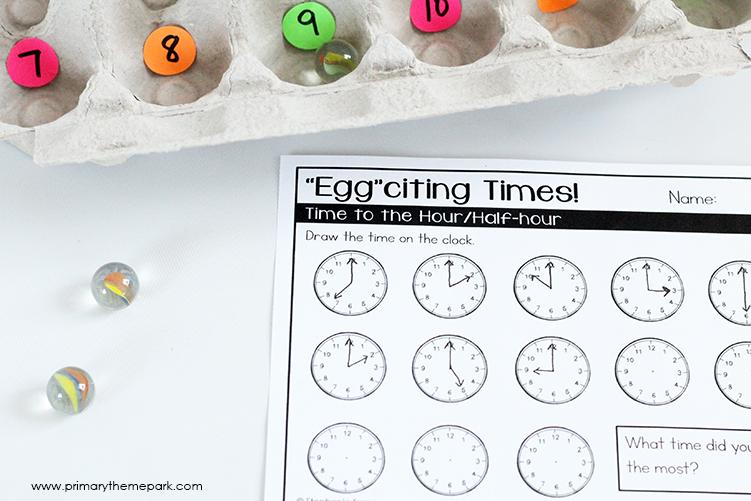### Time to the Hour

Need: 1 marble or small round object

Draw/show the time on a clock.  Example: 3 is 3 o’clock (3:00)

## Egg Carton Math Games Using Numbers 0-9

Need: 2 marbles or small round objects

Make a two-digit number with the numbers the marbles land on. Shake and repeat to make another two-digit number.  Add the two-digit numbers together. Example: 52+16=68.

### Represent Numbers to 120

Need: 2 marbles or small round objects

Make a two-digit number with the numbers the marbles land on. Draw a picture or use tally marks to show the amount.

### Count to 120

Need: 2 marbles or small round objects

Make a two-digit number with the numbers the marbles land on.  Record the number and count on the next three numbers. Example:  26…27…28…29.

### Place Value: Tens and Ones

Need: 2 marbles or small round objects

Make a two-digit number with the numbers the marbles land on. Record how many tens and ones and write the number in expanded form. Example: 26, 2 tens 6 ones, 20+6=26.

### Place Value: Two-digit Numbers

Need: 2 marbles or small round objects

Make 2 different two-digit numbers with the numbers the marbles land on. Example: 24 and 42 using the numbers 2 and 4.

### Compare Two-digit Numbers

Need: 2 marbles or small round objects

Make a two-digit number with the numbers the marbles land on. Shake and repeat to make another two-digit number. Compare the two numbers using > , < , or =. Example: 25 < 39.

### Find 10 More or Less

Need: 2 marbles or small round objects

Make a two-digit number with the numbers the marbles land on.  Find 10 more or 10 less than the number. Example: 25, 10 more is 35, 10 less is 15.

### Make 10

Need: 1 marble or small round object

Add an amount to the number to equal 10. Example:  Land on 6… 6 + ? =10.  6+4=10.

## Egg Carton Math Games Using Special Objects or Numbers

### Subtract Multiples of 10

Egg Carton Spaces: Multiples of 10 from 10 to 90

Need: 2 marbles or small round objects

Subtract the smaller number from the larger number to find the difference.  Example: 80-30=50.

### Graphing

Egg Carton Spaces:  colors red, yellow, green, and blue

Need: 1 marble or small round object

Shade the color on the graph. Shake and repeat a set number of times or until one color reaches the top of the graph.

### Shapes

Egg Carton Spaces:  names of various shapes

Need: 1 marble or small round object

Game 1: Draw the shape it lands on.

Game 2: Draw the shape it lands on and then use that shape to create a drawing.

### Fractions

Egg Carton Spaces:  a fraction in word and/or number form in each egg space (whole or 1, half or ½, fourth or ¼ )

Need: 1 marble or small round object

Shade a circle, square, or rectangle according to the fraction it lands on.

### Money

Egg Carton Spaces:  Coin amounts (\$0.01, \$0.05, \$0.10, \$0.25)

Need: 1 marble or small round object

Game 1:  Color in the coin that matches the amount.

Challenge: Use 2+ marbles or small round objects.  Add together the amounts to find the sum of money.

# FREE Range Eggs Product!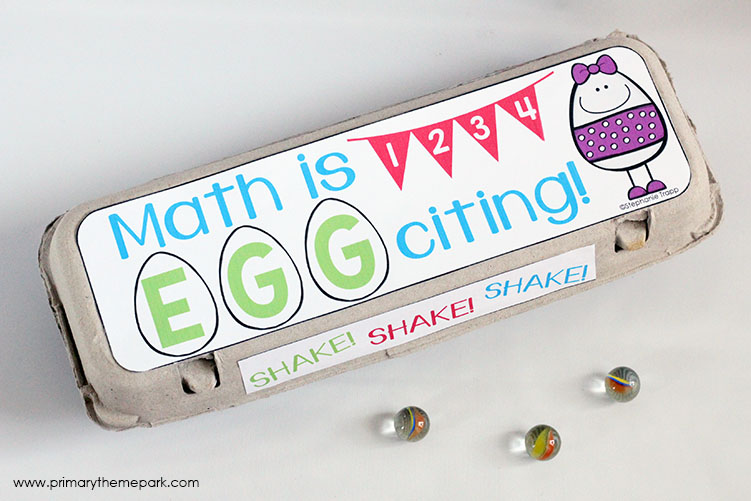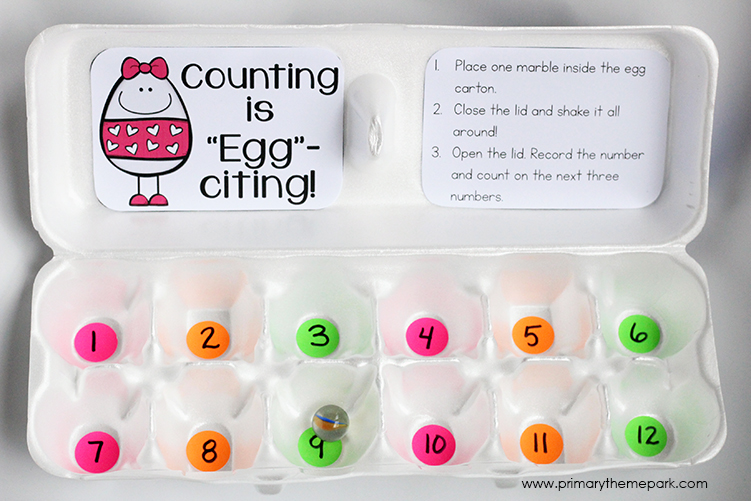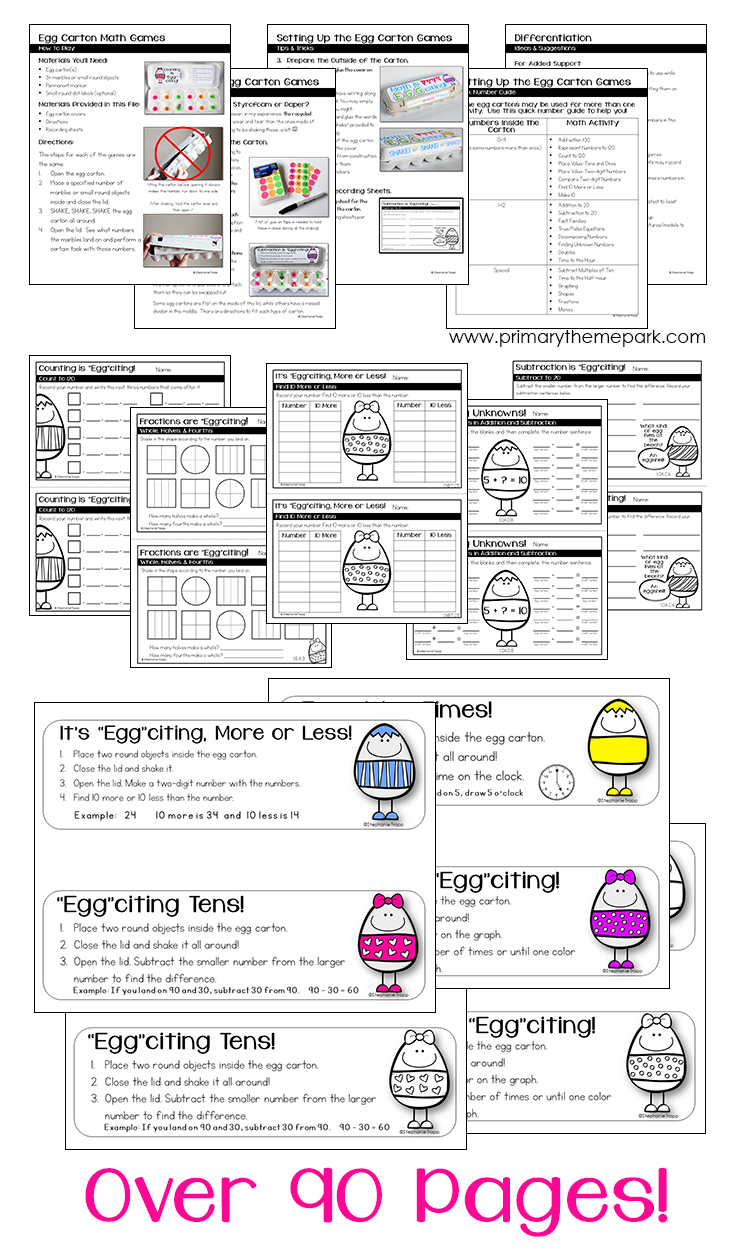Everything you need to create all 22 games, including teacher and student directions, recording sheets, and egg carton covers are a FREE gift from me as a thank you for signing up to my email list.  All you need is an egg carton, some marbles or small round objects, and just a few minutes of set up!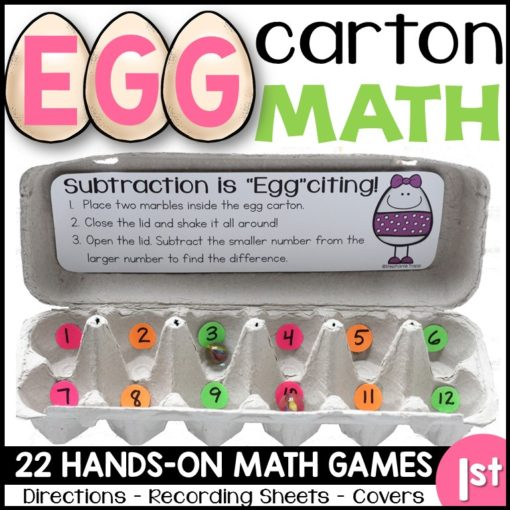## Time Activities for First Grade

1.Robyn Birkenholz says:

I have been using egg cartons for years in my math classroom. They are fabulous.

2.Kim says:

I teqch k-3 at a womens prison – anxious to use these

3.Megan says:

Wonderful ideas!!

4.Eli says:

maravillosas actividades con un material muy fácil de adquirir. Mil gracias por compartir de manera gratuita.

5.Tahani says:

Amazing resource! Can’t thank you enough

6.Stacy Swanson says:

Love these ideas!!